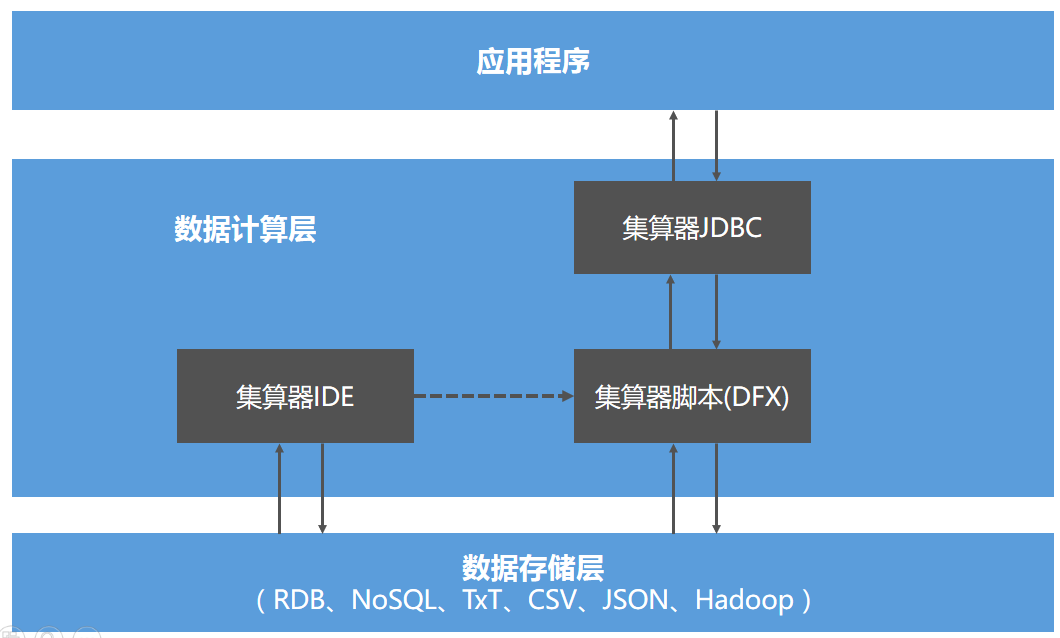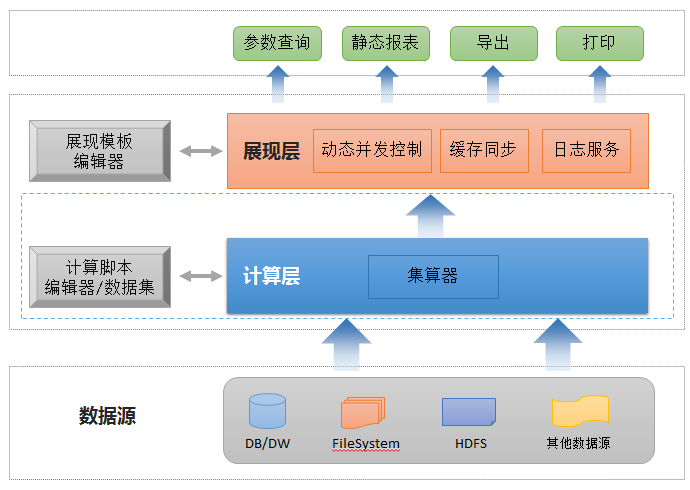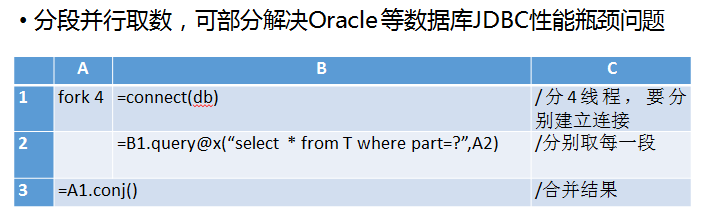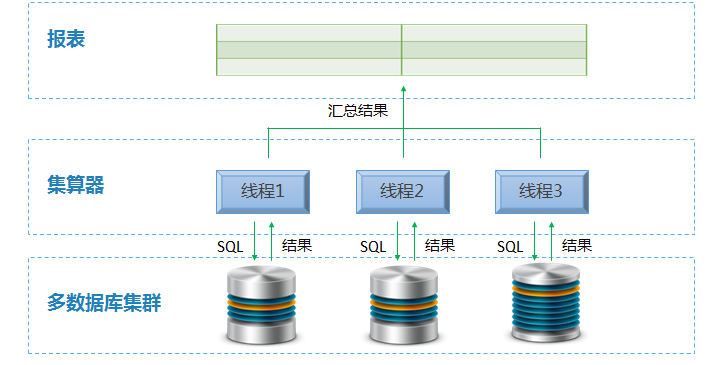# 集算器：解决计算难题的利器

## 集算器

### 相关技术

python(pandas)/R的定位是数学风格的统计分析，虽然提供了dataframe对象用于处理结构化数据，但并不专业，对于外存计算则没有提供直接支持。集算器则是专业的结构化数据处理语言，提供了内存的序表对象（功能相当于dataframe的超集）以及外存的游标对象，能方便编写多线程并行计算，涉及多样性数据源（xls,json,mongodb等）时也有更简单的配置和使用方法。

### 应用结构Java类库

## 结构化数据计算

### 集合化

SQL就没有这个问题，可以简单地写sum(x),sum(x*x),sum(abs(x))。SQL允许用表达式定义一个临时函数，并作为参数传递给某个方法。这个概念在业界的术语叫做lambda语法，这是集合化程序设计语言必须有的机制。

SQL满足集合化语言的基本特征，也因此是目前最常用的结构化数据运算语言。

 A 1 =file(“D.csv”).import@tc(name,sex,age) 2 =A1.select(sex==”M”&&age>=25||sex==”F”&&age>=23) 过滤 3 =A2.sort(name) 排序 4 =A2.groups(sex;avg(age):age) 分组汇总，产生新数据结构 5 =A2.id(left(name,1)) 唯一值

直接集合运算：

 A 1 =file(“T1.txt”).import@ti(id) 2 =file(“T2.txt”).import@ti(id) 3 =A1^A2 交集，即T1和T2共有的值 4 =A1\A2 差集，即T1中有而T2中没有的值

### 离散性

 A 1 =employee.select@1(name=="张三") 2 =employee.select@1(name=="李四") 3 =A1.age-A2.age 4 =A1.salary-A2.salary

 A 1 =agent.sort(amount:-1).to(agent.len()*0.1) 取前10% 2 =A1.run(amount=amount*1.05) 奖励销售额

SQL没有游离记录数据类型，字段的取值当然也不能是另一条记录。SQL采用外键来表示记录之间的引用，关联表较多的时候，JOIN语法写起来非常复杂、容易出错，可读性也很差。如果字段的取值可以是另一条记录，则可以简单地把外键对应的记录看成当前记录的字段，只是这个字段还有子字段。Java等高级语言的对象引用机制就是这样，无论有多少层关联，代码都会很清晰。

 A 1 =file(“D.csv”).import@tc() 2 =file(“P.txt”).import@t(id,area) 3 =A1.switch(aid,A2:aid) 建立外键引用 4 =A3.select(aid.area==”Beijing”) 用外键引用记录的字段过滤

SQL缺乏离散性还有技术方面的的历史原因。在发明SQL的70年代，计算机的内存太小，而在外存实现指针式引用的成本很高，一定要实现，针对于当时还不算复杂的业务需求就不划算了。而40年后的今天，硬件能力的提升已经使离散性不再那么困难，而且业务需求的复杂度也完全不同了。

### 更彻底的集合化

SQL是集合化的语言，但集合化并不够彻底，彻底的集合化需要离散性来支持。

 A 1 =db.query("select * from R") 2 =A1.group(student).select(~.sum(score)>=500).conj()

SQL没有集合数据类型，离散性也不好，无法提供返回结果是记录引用集合的聚合函数，这种运算就需要子查询等繁琐的方式，经常还会导致大排序而损失性能。

SQL可以用窗口函数生成组内排序序号，并取出所有序号为1的记录，但窗口函数是在结果集上再计算的，因而必须用子查询再过滤的形式，写法有些复杂。而不支持窗口函数的数据库写起来就会更困难了。

 A 1 =db.query("select * from L order by login") 2 =A1.group@1(user)

 A 1 =db.query("select * from S") 2 =A1.groups(code;top(2,-date)) 最后2个交易日的数据 3 =A2.new(code,#2(1).cp-#2(2).cp:price-rises) 计算涨幅

 A 1 =db.query("select * from S order by date desc") 2 =A1.groups(code;top(2,0)) 直接取前2条 3 =A2.new(code,#2(1).cp-#2(2).cp:price-rises)

SQL的集合化不彻底，从明细到汇总很容易，反过来就困难很多，要将记录数变多一般是和一个序号表JOIN或用递归查询，思路很不直接。而集算器则按常规思路写出来即可：

 A 1 =db.query("select * from I") 2 =A1.news(periods;no,~:seq,after@m(start,~-1):date,sum/periods:amount)

### 有序计算

MySQL不支持窗口函数，但支持在SQL中使用变量，可以引用到前面的行，但无法引用到后面的行。

 A 1 =db.query("select * from S order by prod,month") 2 =A1.select(if(prod==prod[-1],sales/sales[-1])>1.1)

 A 1 =db.query("select * from S order by product,month") 2 =A1.derive(if(prod==prod[-1]&&prod==prod,sales{-1:1}.avg()):moving-avg)

 A 1 =db.query("select * from E order by seq") 2 =A1.select(time!=max(time{:0})||time!=min(time{0:})) 和前后所有记录对比

SQL只提供与次序无关的等值分组，但有时分组的键值并不能在每条记录中找到，而是和记录的次序有关，这种情况，用SQL又需要使用窗口函数（或其它更麻烦的手段）制造出序号才能实现。

 A 1 =db.query("select * from B order by month") 2 =A1.group@o(income>expense).select(~.income<~.expense && ~.len()>=3).conj()

group@o表示在分组时只比较相邻记录，如果相邻值发生变化则会分出一个新组。这样就可以根据收入支出的比较把收支记录分成赢利、亏损、赢利、…这样的组，然后取出其中亏损且成员不少于3的组再合并起来。

 A 1 =db.query("select * from B order by month") 2 =A1.group@i(income

group@i将在条件变化时分出一个新组，即收入降低时。

 A 1 \$select S,E from T order by S 2 =A1.select(E>max(E{:-1})) 去除被包含的条目 3 =A2.run(max(S,E[-1]):S) 去除重叠时间段 4 =A2.sum(interval@s(max(S,E[-1]),E)) 计算总时长 5 =A2.run(if(S

 A 1 =db.query@i("select price from T order by price") 2 =A1([(A1.len()+1)\2,A1.len()\2+1]).avg()

 A 1 =db.query@i("select time from E order by time") 2 =A1.group((#-1)\2).sum(interval@s(~(1),~(2))

#表示记录序号，group((#-1)\2)即将数据每两个分成一组，然后针对每组计算时长再合计即可。

 A 1 =db.query("select * from S order by date") 2 =A1.pselect@a(cp>100).select(~>1) 3 =A2.new(A1(~).date:date,A1(~).cp-A1(~-1).cp:price-rises)

pselect函数将返回满足条件的成员位置，使用这些位置就可以方便地计算涨幅，而不必像使用窗口函数时事先计算出所有涨幅再过滤。

 A 1 =db.query("select * from T order by date") 2 >start=A1(1).date 3 =interval(start,A1.m(-1).date)\7+1 计算总周数 4 =A1.align@a(A2,interval(start,date)\7) 按周分组，可能有空集 5 =A4.new(#:week,acc[-1]+~.sum(amount):acc) 汇总并计算累计

### 游标技术

 A 1 =file(“Products.txt”).import().primary@i(id) 读入商品列表并建立索引 2 =file(“Sales.txt”).cursor() 建立游标，准备遍历 3 =A2.select(quantity<=10) 过滤，仍返回游标 4 =A3.switch(productid,A1:id) 建立连接指针，仍返回游标 5 =A4.groups(;sum(quantity*productid.price)) 求和汇总

A2建立原始游标，A3在A2基础上过滤，A4在A3基础上建立连接指针，A5最后针对A4计算出汇总结果。实质的数据遍历动作只在A5中的计算过程时才实施，A2,A3,A4仅仅是记录游标信息，并不实际运算。但这种多级游标的代码看起来像是一步步地处理游标，理解和编写都很简单。
SQL型的数据库都提供了游标，但只有简单的fetch数据功能，没有封装运算，更没有多级游标，要在这种游标基本的完成复杂运算会相当麻烦。

 A B 游标处理程序sub.dfx 1 =file(“Sales.txt”).cursor() 2 for A1,1000 … 每次取出1000条记录处理 … … … return … 处理后的结果返回

 A 主程序 1 =cursor("sub.dfx") 使用子程序 2 =A1.fetch( 100 ) 当作普通游标使用

 A B 1 =file(“userlogs.txt”).cursor() 按用户id排序的源文件 2 for A1;id … 从游标中循环读入数据，每次读出一组id相同 3 … 处理计算该组数据

### 动态列能力

SQL是解释执行的语言，可以临时生成动态的数据结构，这方面问题不大。不过，SQL认为列是数据的属性，是静态的，没有提供针对列上的集合运算。这样在我们事先不知道列的信息或列很多需要通用方式处理时就会显得很麻烦。

 A 1 =db.query("select * from PE") 2 =A1.conj(~.array().to(2,)) 从第2字段的各项目成绩合并起来 3 =A2.groups(~:grade;count(1):count) 分组汇总

 A B C 1 =db.query("select * from R") 2 =A1.pivot@r(student,semester;subject,score) 3 =A2.pivot(student,subject;semester,score)

A2将列转成行，A3再将行转成列，从而实现双向转置。

 A B C 1 =db.query("select * from R order by student,semester") 2 =create(student,subject,\${A1.id(semester).string()}) 3 for A1.group(student) for 3,A1.fno() =A3.field(B3) 4 >A2.record(A1.student|A2.fname(B3)|C3) 5 return A2

A2中先用宏生成目标结果集，再在A3-C4的循环中将数据变换后插入到结果集，这是集算器实现转置任务的标准流程，分步运算使代码更清晰易懂。这个方案也可用于静态或单向转置，代码更简单。集算器的列访问机制和动态语言的灵活性，使得各种转置，静态或动态、行转列还是列转行以及双向同时，都可以采用一致的方案实现。

 seq acount state date 1 A over 2014-1-4 2 A OK 2014-1-8 3 A lost 2014-3-21 …

 account 1 2 3 4 5 6 7 8 9 … 3 1 A over over over over OK OK … OK ...

 A B 1 =db.query("select * from T where year(date)=? and month(date)=?",2014,1) 2 =create(account,\${to(31).string()}) 3 for A1.group(account) =31.(null) 4 >A3.run(B3(day(date))=state) 5 >B3.run(~=ifn(~,~[-1]) 6 >A2.record(A3.account|B3) 7 return A2

### 准结构化数据

20010-8-13 991003 3166.63 3332.57  3166.63  3295.11
2010-8-10 991003 3116.31 3182.66  3084.2   3140.2
……

string()函数可将集合按指定分隔符再拼成字串。

COOP:166657,'NEW IBERIA AIRPORT ACADIANA REGIONAL LA US',200001,177,553
……

 A 1 =file("T.txt").read@n() 2 =A1.group(mid(~,pos(~," US'")-2,2):state;~:data) 3 >A2.run(file(state+".txt").export(data))

 A B 1 =file(“S.log”).read@n() 2 =create(…) 建立目标结果集 3 for A1.group((#-1)\3) … 按行号分组，每3行一个单位 … … 从A3（这3行）中解析出字段值 … >A2.insert(…) 插入到目标结果集 … >file(“T.txt”).export(A2) 写出结果

 A B 1 =file(“S.log”).cursor@si() 创建游标用流式读入文件 2 =file(“T.txt”) 结果文件 3 for A1,3 … 每读入3行执行一轮循环 … … 从A3（这3行）中解析出字段值 … >A2.export@a(...) 追加写到文件中

 3 for A1.group@i(~==”---start---”) 出现---start---时会产生一个新分组

 3 for A1;~==”---start—“:0 出现---start---时另起一轮循环

 3 for A1.group@o(left(~,6)) 前6个字符变化时产生一个新组 3 for A1;left(~,6) 前6个字符变化时另起一轮循环

grep是常用的unix命令，但有些操作系统下没有，且在程序中实现也不简单。集算器提供了文件系统的遍历功能，结合文本计算能力，只要两句代码就能完成。

WordCount是著名的练习题，集算器提供了words()函数将串拆分成单词，只要一句就可以完成这个运算。

json/xml
Java有足够多的类库用于解析和生成json/xml，但缺乏后续计算能力。集算器支持多层结构数据，可以不丧失信息地将json/xml解析成可计算的内存数据表进一步处理。

{
“order”:[
{
“client”:”北京润乾软件”,
“date”:”2015-6-23”,
“item” : [

“product”:”HP笔记本”,
“number”:4,
“price”:3200
},
{
“product”:”DELL服务器”,
“number”:1,
“price”:22100
}]
},…]
}

 A 1 =file(“data.json”).read().import@j().order 2 =A1.new(#:orderid,client,date) 3 =A1.news(item;A1.#:orderid,#:seq,product,number,price) 4 >db.update@i(A2,order) 5 >db.update@i(A3,ordedetail)

## 大数据计算技术

### 大数据技术

SQL

SQL，或者说关系代数，没有办法表达这种运算，只能寄希望于数据库厂商的优化工作了。SQL这种问题很多，下面会逐步碰到。

### 内存计算

 A 1 =file(“Products.txt”).import() 读入商品列表 2 =file(“Sales.txt”).import() 读入销售记录 3 >A2.switch(productid,A1:id) 建立指针式连接，把商品编号转换成指针 4 =A2.sum(quantity*productid.price) 计算销售金额，用指针方式引用商品单价

 集算器 Oracle 单表无连接 0.57s 0.623s 五表外键连接 2 .3s 5.1s

Oracle没有纯内存的方案，多次运行后，Oracle会将数据缓存到内存中，但仍然可能会有些额外处理，所以横向绝对值比较意义不大，但数值的差异倍数有意义，在无外键连接时，集算器与Oracle性能相差不大，但有了连接运算后，集算器比Oracle快了一倍多。

 A 1 =file(“Products.txt”).import().primary@i(id) 读入商品列表并建立索引 2 =file(“Sales.txt”).cursor() 建立销售记录游标，准备遍历 3 =A2.switch(productid,A1:id) 在游标上建立连接指针，准备遍历 4 =A3.groups(;sum(quantity*productid.price)) 遍历计算销售金额，仍可用指针引用

 A 1 =file(“Products.txt”).import() 读入商品列表 2 =file(“Sales.txt”).cursor() 根据已序号化的销售记录建立游标 3 =A2.switch(productid,A1:#) 用序号定位建立连接指针，准备遍历 4 =A3.groups(;sum(quantity*productid.price)) 计算结果

SQL在理论上延用了数学上的无序集合概念，表中记录没有次序，即使人为地使用序号来表示外键了，数据库仍然会使用HASH JOIN算法，仍要计算和比较HASH值，事先费事准备数据也不能起到提高性能的作用。

 A 1 =file(“Products.dat”).create().primary@i(id) 根据文件创建字节表并建索引 2 =file(“Sales.dat”).cursor@b() 建立销售记录游标，准备遍历 3 =A2.switch(productid,A1) 和普通内存表一样使用 4 =A3.groups(;sum(quantity*productid.price)) 计算方案也一样

 A B 1 =file(“Sales.dat”).create() 源数据读入成字节表 2 fork 4 =A1.cursor(A2:4) 分作4段并行，分别建立内存游标 3 =B2.groups(;sum(amount):a) 遍历游标计算amount之和 4 =A2.conj().sum(a) 汇总每个线程的结果

 A 1 =file(“Sales.txt”).import() 取出数据 2 =A1.select@m(amount>1000) 并行过滤 3 =A2.sort@m(amount:-1) 并行排序

@m选项将自动根据系统配置决定并行的线程数量。不过，内置并行无法保证记录的取用次序，在涉及有序计算时就不能用了。

### 外存计算

 A 1 =file(“data.txt”).cursor() 2 >A1.groups@x(;count(1);n) 绑定后计算的汇总，在遍历时再计算 3 =A1.sortx(key) 排序，遍历过程中处理A2的绑定计算 4 =A1.v(n).#1 取出绑定计算的结果，即总记录数 5 =A3.skip((A4-1)\2).fetch@x(2-A4%2).avg(key) 取出中位数记录并计算中位数

 A B 1 =file(“data.txt”) 源文件 2 fork 4 =A1.cursor@t(amount;A2:4) 分作4段并行，分别建立游标 3 =B2.groups(;sum(amount):a) 遍历游标计算amount之和 4 =A2.conj().sum(a) 汇总每个线程的结果

 A 1 =file(“data.txt”).cursor@tm(amount) 定义并行取数的游标 2 =A1.groups(;sum(amount))._1 遍历游标并汇总amount列

 A B 1 fork 4 =connect(db) 分4线程，要分别建立连接 2 =B1.query@x(“select * from T where part=?”,A2) 分别取每一段 3 =A1.conj() 合并结果

 A 1 =file(“data.txt”).cursor@t() 定义文本文件游标 2 =file(“data.bin”).export@z(A1) 写成可分段的二进制文件

 A B 1 =file(“data.bin”) 源文件 2 fork 4 =A1.cursor@b(amount;A2:4) @b表示二进制文件，其它参数相同 3 =B2.groups(;sum(amount):a) 后续计算语法完全相同 4 =A2.conj().sum(a)

 A 1 =file(“data.txt”).cursor@t() 原文本文件 2 =10.(file(“col”/~/”.bin”)) 产生10个列的对应存储文件 3 >A2.export@z(A1) 将原数据写成可分段的多个列存文件

 A 1 =[1,3].(file(“col”/~/”.bin”)) 使用第1,3列 2 =A1.cursor() 定义列存游标 3 =A2.groups(;sum(col1+col3):all)._1 使用游标计算合计

 A B 1 =[1,3].(file(“col”/~/”.bin”)) 定义列存文件组 2 fork 4 =A1.cursor(amount;A2:4) 并行和游标代码与行存相同 3 =B2.groups(;sum(amount):a) 后续计算语法完全相同 4 =A2.conj().sum(a)

 A 1 =file(“Order.txt”).cursor@t() 订单游标，按订单id排序 2 =file(“Detail.txt”).cursor@t() 订单明细游标，也按订单id排序 3 =join@x(A1:O,id;A2:D,id) 有序归并连接，仍返回游标 4 =A3.groups(O.area;sum(D.amount)) 按地区分组汇总金额，地区字段在主表中，金额字段在明细子表中

 A 1 =file(“userlog.txt”).cursor@t() 原始数据游标 2 =A1.sortx(id) 按id排序并返回游标 3 >file(“userlog.dat”).export@z(A2;id) 写成按id分段的二进制文件

 A B C 1 =file(“userlog.dat”) 对id有序的数据文件 2 fork 4 =A1.cursor@b(;A2:4) 分段时保证同一组id不会被拆开 3 for B2;id … 后续计算语法相同 4 …

 A 1 =file(“Order.txt”).cursor@t() 2 =A1.sortx(id) 按id排序 3 >file(“Order.dat”).export@z(A2;id) 写成按id分段的二进制文件 4 =file(“Detail.txt”).cursor@t() 5 =A4.sortx(id) 按id排序 6 >file(“Detail.dat”).export@z(A2;id,file(“Order.dat”),id ) 和Order.dat同步按id分段

 A B 1 =file(“Order.dat”) 对id有序的数据文件 2 =file(“Detail.dat”) 同步分段的数据文件 3 fork 4 =A1.cursor@b(;A3:4) 分段时保证同一组id不会被拆开 4 =A2.cursor@b(;A3:4) 同步分段 5 =join@x(B3:O,id;B4:D,id) 归并 6 =B5.groups(O.area;sum(D.amount)) 汇总 7 =A3.conj().groups(#1;sum(#2)) 合并后再汇总

### 集群计算

 A B 1 =4.(“192.168.0.”/(10+~)/”:1234”) 节点机列表，4个 2 fork to(8);A1 到节点机上执行，分成8个任务 3 =hdfsfile(“hdfs:\\192.168.0.1\persons.txt”) HDFS上的文件 4 =B3.cursor@t(;A2:8) 分段游标 5 =B4.select(gender==’M’).groups(;count(1):C) 过滤并计数 7 =A2.conj().sum(C) 汇总结果A B C 1 =4.(“192.168.0.”/(10+~)/”:1234”) 节点机列表，4个 2 fork to(8);A1 到节点机执行 3 =file(“person.txt”,A2) A2为数据区号 4 if !B3.exists() end “data not find” 找不到返回错误再分配 5 =B3.cursor() 6 =B5. select(gender==’M’).groups(;count(1):C) 计算 7 =A2.conj().sum(C) 汇总

 A 主程序 1 =8.(“192.168.0.”/(10+~)/”:1234”) 节点列表，共8个 2 =hosts(A1,to(4),”init.dfx”) 在其中寻找4个节点加载内存数据 3 =callx@a(“sub.dfx”,to(4);A2) 调用这些节点上程序计算 4 =A3.sum() 汇总结果
 A 节点初始化程序init.dfx 1 =file(“Product.txt”,z).import() 读入第z分区的数据 2 >env(T,A1) 将数据记入环境 3 >zone(z) 登记本节点的分区
 A 节点程序sub.dfx 1 =env(T) 从环境中取出数据 2 =A1.count(gender==’M’) 过滤并计数 3 return A2 返回结果

 A 主程序 1 =8.(“192.168.0.”/(10+~)/”:1234”) 节点机列表，共8个 2 =hosts(A1,to(4),”init.dfx”) 选择4个节点加载内存维表 3 =callx(“sub.dfx”,8*A2,to(8);A1) 调用节点程序计算，传入内存维表节点 4 =A3.sum() 汇总结果
 A 节点初始化程序init.dfx 1 =file(“Product.txt”,z).import() 读入第z分区的维表 2 >env(T,A1) 将数据记入环境 3 >zone(z) 登记本节点的分区
 A B 节点程序sub.dfx 1 =file(“Sales.txt”,z) z区事实数据 2 if !A1.exists() end “data not find” 找不到返回错误 3 =createx(T,h) 基于节点组h上变量T建立集群维表 4 =A1.cursor() 5 =A4.join(productid,A3,price) 与集群维表A3做连接计算 6 =A5.sum(quantity*price) 合计 7 return A6 返回结果

JOIN总结

SQL对于JOIN的定义就是简单的笛卡尔积再过滤，定义简单的好处在于描述面非常广泛，很多关联运算可以看成是SQL的JOIN，针对这种JOIN研究的成果适应面非常广。但太简单的定义在实施运算时能够利用的特征太少，运算优化就不好做了。相反地，把定义复杂化，分各种情况讨论，虽然描述广泛度下降了，但可以根据情况的特征进行优化。

SQL的JOIN也是类似，在进一步细化分类后，可以针对各类任务的特征分别提出更优化的算法。

## 报表型应用协助

### 引入计算引擎

“零编码开发报表”是许多报表工具厂商都喊过的口号，但现实并不是这么简单，虽然确实有不少简单报表可以拖拖拽拽完成，但仍然有相当数量的报表必须经过编码才能完成。### 提升报表运算性能